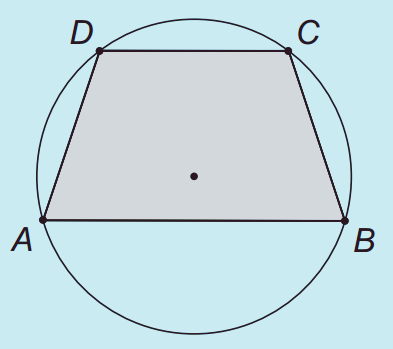# A geometry problem by Jádson Bráz

Geometry Level 2In the following figure, $ABCD$ is a trapezoid inscribed in a circle. The longer base $AB$ has length 16 cm, the shorter base $CD$ has length 10 cm, and the altitude of the trapezoid has length 9 cm. What is the radius of the circle, in centimeters?

×